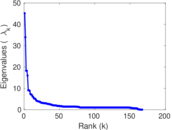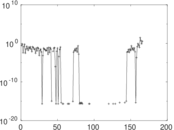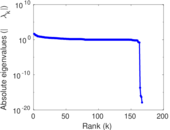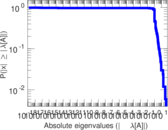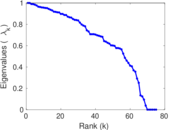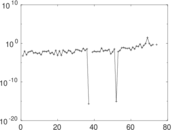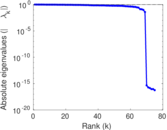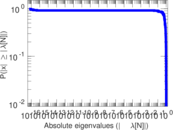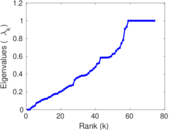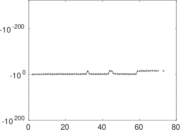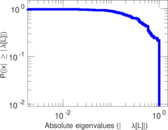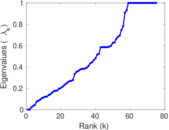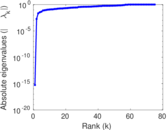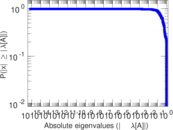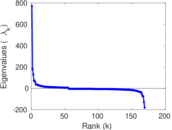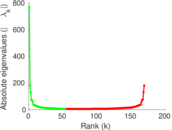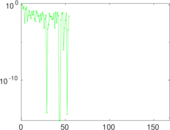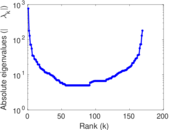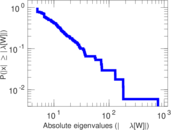# Wikibooks edits (cy)

This is the bipartite edit network of the Welsh Wikibooks. It contains users and pages from the Welsh Wikibooks, connected by edit events. Each edge represents an edit. The dataset includes the timestamp of each edit.

 Code `bcy` Internal name `edit-cywikibooks` Name Wikibooks edits (cy) Data source http://dumps.wikimedia.org/ AvailabilityDataset is available for download Consistency checkDataset passed all tests Category Authorship network Dataset timestamp 2017-10-20 Node meaning User, article Edge meaning Edit Network formatBipartite, undirected Edge typeUnweighted, multiple edges Temporal dataEdges are annotated with timestamps

## Statistics

 Size n = 781 Left size n1 = 169 Right size n2 = 612 Volume m = 1,170 Unique edge count m̿ = 755 Wedge count s = 27,176 Claw count z = 1,404,998 Cross count x = 58,091,812 Square count q = 509 4-Tour count T4 = 114,958 Maximum degree dmax = 283 Maximum left degree d1max = 283 Maximum right degree d2max = 45 Average degree d = 2.996 16 Average left degree d1 = 6.923 08 Average right degree d2 = 1.911 76 Fill p = 0.007 299 76 Average edge multiplicity m̃ = 1.549 67 Size of LCC N = 535 Diameter δ = 16 50-Percentile effective diameter δ0.5 = 5.880 07 90-Percentile effective diameter δ0.9 = 9.843 02 Median distance δM = 6 Mean distance δm = 6.287 67 Gini coefficient G = 0.633 395 Balanced inequality ratio P = 0.255 128 Left balanced inequality ratio P1 = 0.183 761 Right balanced inequality ratio P2 = 0.338 462 Relative edge distribution entropy Her = 0.850 378 Power law exponent γ = 5.080 16 Tail power law exponent γt = 2.641 00 Tail power law exponent with p γ3 = 2.641 00 p-value p = 0.000 00 Left tail power law exponent with p γ3,1 = 1.991 00 Left p-value p1 = 0.209 000 Right tail power law exponent with p γ3,2 = 4.581 00 Right p-value p2 = 0.065 000 0 Degree assortativity ρ = −0.185 673 Degree assortativity p-value pρ = 2.775 62 × 10−7 Spectral norm α = 45.188 5 Algebraic connectivity a = 0.001 823 66 Spectral separation |λ1[A] / λ2[A]| = 1.331 30 Controllability C = 448 Relative controllability Cr = 0.578 811

## Plots

### Fruchterman–Reingold graph drawing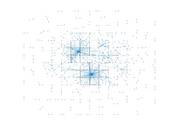### Degree distribution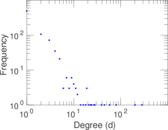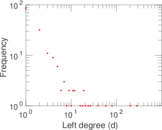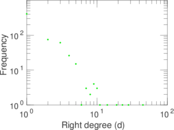### Cumulative degree distribution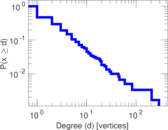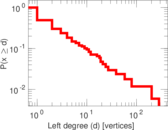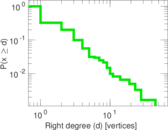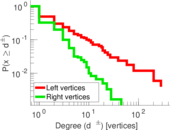### Lorenz curve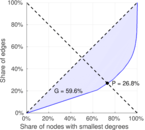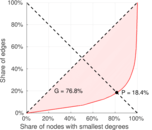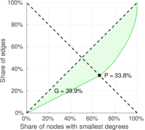### Spectral distribution of the adjacency matrix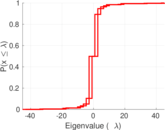### Spectral distribution of the normalized adjacency matrix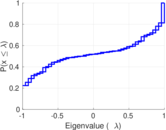### Spectral distribution of the Laplacian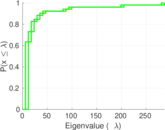### Spectral graph drawing based on the adjacency matrix### Spectral graph drawing based on the Laplacian### Spectral graph drawing based on the normalized adjacency matrix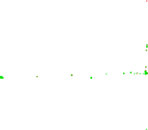### Degree assortativity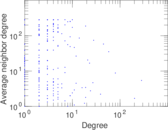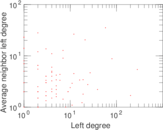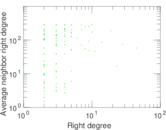### Zipf plot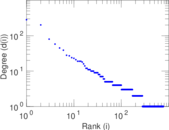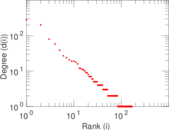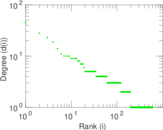### Hop distribution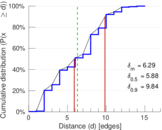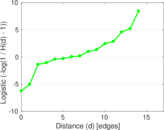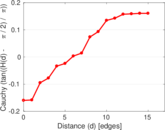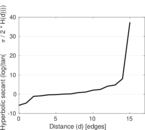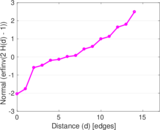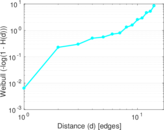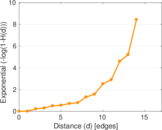### Double Laplacian graph drawing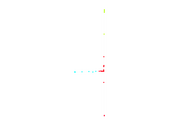### Delaunay graph drawing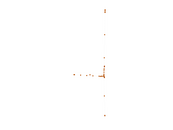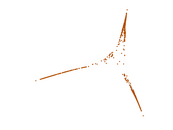### Edge weight/multiplicity distribution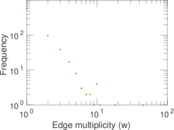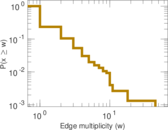### Temporal distribution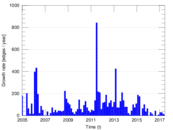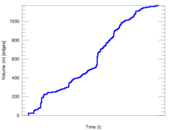### Temporal hop distribution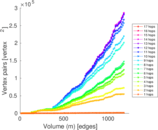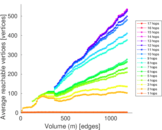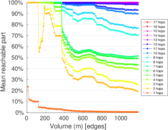### Diameter/density evolution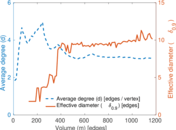### Matrix decompositions plots